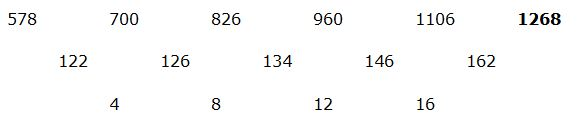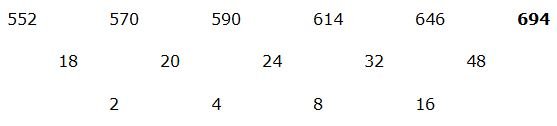# CWC/FCI Prelims 2019 – CWC/FCI Quantitative Aptitude Questions (Day-49)

Dear Readers, Exam Race for the Year 2019 has already started, CWC/ FCI will be the most expected exam among the aspirants. Preparation and performance both requisite to clear CWC/FCI Exam. To enrich your preparation here we are providing a new series of Practice Questions on CWC/ FCI Quantitative Aptitude Section. Aspirants, practice these questions on a regular basis to improve your score in the aptitude section. Start your effective preparation from the right beginning to get success in the upcoming CWC/FCI Exam.

[WpProQuiz 6035]

## CWC/FCI Quantitative Aptitude Questions (Day-49)

Directions (Q. 1 – 5) what value should come in place of (?) in the following number series?

1) 578, 700, 826, 960, 1106, ?

a) 1416

b) 1154

c) 1020

d) 1268

e) 1342

2) 1971, 1970, 984, 327, ? , 15.15

a) 80.75

b) 95.25

c) 62.5

d) 68.75

e) 86.25

3) 552, 570, 590, 614, 646, ?

a) 642

b) 660

c) 694

d) 718

e) 736

4) 73, 37, 38, 58.5, 119, ?

a) 275

b) 320

c) 225

d) 300

e) 350

5) 384, 204, 112, 64, ?

a) 26

b) 38

c) 32

d) 30

e) 24

Directions (Q. 6 – 10): Study the following table carefully and answer the given questions:

The following table shows the total number of people working in various departments of different organizations in particular city.

 Department Organizations A B C D E Production 875 520 750 640 725 IT 915 852 743 680 570 Accounts 595 925 742 678 800 Marketing 690 875 627 773 945 Finance 886 914 755 685 500

6) Total number of employees working in Accounts department is approximately what percentage of total number of employees working in marketing department of all the given organizations?

a) 84 %

b) 96 %

c) 75 %

d) 108 %

e) 117 %

7) Find the difference between the total number of employees working in organization C to that of D of all the given department together?

a) 154

b) 149

c) 173

d) 161

e) None of these

8) Total number of employees working in IT and Finance of Organization A, C and E together is approximately what percentage more/less than the total number of employees working in Production and Marketing of Organization B, D and E together?

a) 2 % more

b) 11 % less

c) 2 % less

d) 11 % more

e) 16 % less

9) Find the ratio between the total number of employees working in Organization B to that of E of all the given department together?

a) 681 : 590

b) 789: 512

c) 871: 793

d) 879: 198

e) None of these

10) Which of the following Organization has highest number of employees?

a) Organization A

b) Organization B

c) Organization C

d) Organization D

e) Organization EThe difference of difference is, 4, 8, 12, 16,…

The pattern is,

(1971 – 1)/1 = 1970

(1970 – 2)/2 = 984

(984 – 3)/3 = 327

(327 – 4)/4 = 80.75

(80.75 – 5)/5 = 15.15The difference of difference is, 21, 22, 23, 24,….

The pattern is, *0.5 + 0.5, *1 + 1, *1.5 + 1.5, *2 + 2, *2.5 + 2.5,….The difference is, ÷ 2 + 2

Directions (6-10):

Total number of employees working in Accounts department

= > 595 + 925 + 742 + 678 + 800 = 3740

Total number of employees working in marketing department

= > 690 + 875 + 627 + 773 + 945 = 3910

Required %= (3740/3910)*100 = 95.65 % = 96 %

The total number of employees working in organization C

= > 750 + 743 + 742 + 627 + 755 = 3617

The total number of employees working in organization D

= > 640 + 680 + 678 + 773 + 685 = 3456

Required difference = 3617 – 3456 = 161

Total number of employees working in IT and Finance of Organization A, C and E together

= > 915 + 743 + 570 + 886 + 755 + 500 = 4369

Total number of employees working in Production and Marketing of Organization B, D and E together

= > 520 + 640 + 725 + 875 +773 + 945 = 4478

Required % = [(4478 – 4369)/4478]*100 = (109/4478)*100

= > 2.434 % = 2 % less

The total number of employees working in Organization B

= > 520 + 852 + 925 + 875 + 914 = 4086

The total number of employees working in Organization E

= > 725 + 570 + 800 + 945 + 500 = 3540

Required ratio = 4086: 3540 = 681: 590

Total number of employees in Organization A

= > 875 + 915 + 595 + 690 + 886 = 3961

Total number of employees in Organization B

= > 520 + 852 + 925 + 875 + 914 = 4086

Total number of employees in Organization C

= > 750 + 743 + 742 + 627 + 755 = 3617

Total number of employees in Organization D

= > 640 + 680 + 678 + 773 + 685 = 3456

Total number of employees in Organization E

= > 725 + 570 + 800 + 945 + 500 = 3540

Organization B has highest number of employees.

Related Articles: